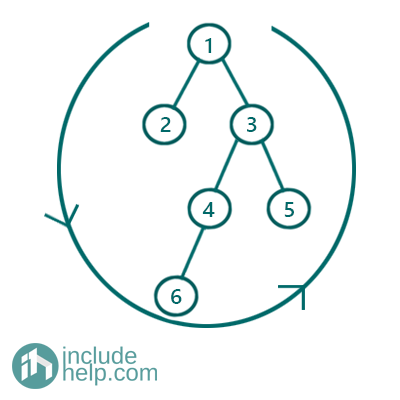# Boundary Traversal of a binary Tree

In this article, we are going to see how to traverse boundary of a tree anti-clock wise which is popularly known as boundary traversal?
Submitted by Radib Kar, on August 01, 2020

We have discussed a lot of traversal techniques already. Here we are going to traverse the boundary of a tree anti-clockwise.Figure 1: Boundary traversal of the tree

Like for the above tree, the boundary traversal for the above tree will be 1, 2, 6, 4, 5, 3

Solution:

We can break the boundary traversal into four parts in order:

1. The root(first one to be printed)
2. The left most nodes of the left subtree except the leaf nodes
3. The leaf nodes
4. The rightmost nodes in the right subtree (in reverse order)

1) Traverse the root

This is as trivial as traversing the root only.

2) Find the left most nodes of the left subtree

If current is not leaf traverse current
To traverse leftmost nodes
If current has left child
Set current=current->left
Else
Set current=current->right
Repeat until current is NULL

3) Find leave nodes

Recursively find the leaf nodes from left to right

4) The rightmost nodes in the right subtree (in reverse order)

We can do similarly as we did in the step 2(Find the left most nodes of the left subtree), but here to maintain the anti-clockwise order, we need traverse in reverse order and thus we need stack to store the traversal.

If current is not leaf store that into stack,
To traverse rightmost nodes
If current has right child
Set current=current->right
Else
Set current=current->left
Repeat until current is NULL
After the loop ends, pop and print the stack

These successfully completes the boundary traversal. Below is the C++ implementation of the above algorithm.

C++ Implementation:

```#include <bits/stdc++.h>
using namespace std;

class TreeNode {
public:
int val;
TreeNode* left;
TreeNode* right;

TreeNode(int v)
{
val = v;
left = NULL;
right = NULL;
}
};

{
if (!root->left && !root->right)
arr.push_back(root->val);
if (root->left)
if (root->right)
}

vector<int> boundaryOfBinaryTree(TreeNode* root)
{
vector<int> arr;
if (!root)
return arr;
arr.push_back(root->val);
if (!root->left && !root->right)
return arr;
TreeNode* leftChild = root->left;
while (leftChild) {
if (!(!leftChild->left && !leftChild->right))
arr.push_back(leftChild->val);

if (leftChild->left)
leftChild = leftChild->left;
else
leftChild = leftChild->right;
}

TreeNode* rightChild = root->right;
stack<int> st;
while (rightChild) {
if (!(!rightChild->left && !rightChild->right))
st.push(rightChild->val);

if (rightChild->right)
rightChild = rightChild->right;
else
rightChild = rightChild->left;
}

while (!st.empty()) {
arr.push_back(st.top());
st.pop();
}

return arr;
}

int main()
{
//Tree is built like the example
TreeNode* root = new TreeNode(1);

root->left = new TreeNode(2);
root->right = new TreeNode(3);
root->right->left = new TreeNode(4);
root->right->right = new TreeNode(5);
root->right->left->left = new TreeNode(6);

cout << "Boundary traversal of the example tree is:\n";

vector<int> arr = boundaryOfBinaryTree(root);
for (auto it : arr)
cout << it << " ";
cout << endl;

return 0;
}
```

Output:

```Boundary traversal of the example tree is:
1 2 6 5 3
```

Languages: » C » C++ » C++ STL » Java » Data Structure » C#.Net » Android » Kotlin » SQL
Web Technologies: » PHP » Python » JavaScript » CSS » Ajax » Node.js » Web programming/HTML
Solved programs: » C » C++ » DS » Java » C#
Aptitude que. & ans.: » C » C++ » Java » DBMS
Interview que. & ans.: » C » Embedded C » Java » SEO » HR
CS Subjects: » CS Basics » O.S. » Networks » DBMS » Embedded Systems » Cloud Computing
» Machine learning » CS Organizations » Linux » DOS
More: » Articles » Puzzles » News/Updates